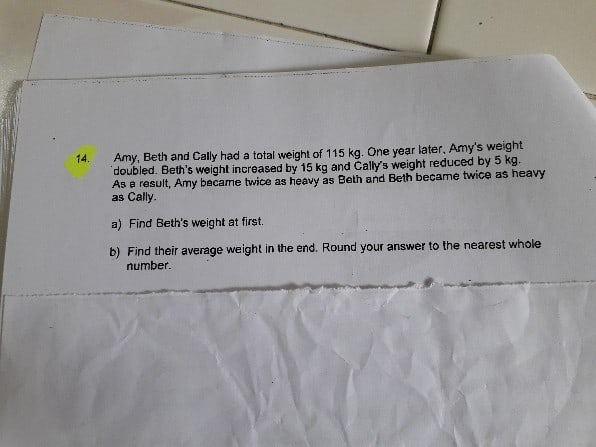# QuestionHelp me to solve this problem

Thank you

At present,

Cally weight = C

Beth weight = 2C

Amy weight = 2B = 4C

one year back,

Cally weight = C + 5

Beth weight  = 2C – 15

Amy weight  =  2C

2C + 2C – 15 + C + 5 = 115

5C -10 = 115

5C = 125

1C = 25

(a) Earlier Cally’s weight = c + 5 = 25 +5 = 30. Beth weight was 2C = 2 x 30 = 60Kg.

(b) current weight

Amy = 100kg, Beth = 50Kg, Cally=25Kg. Average = 58Kg.

0 Replies 0 Likes